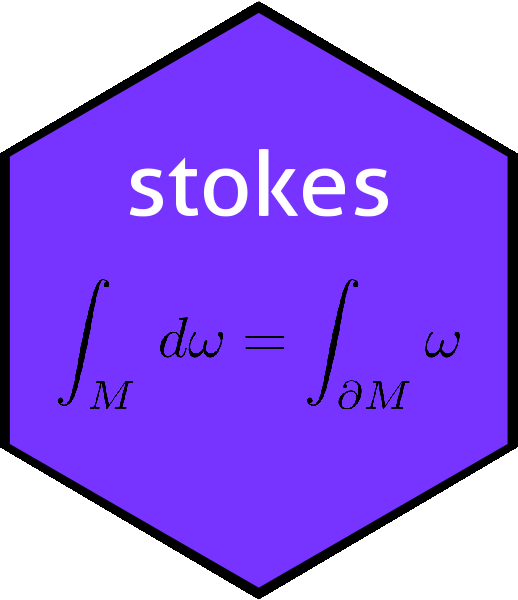# Function vector_cross_product() in the stokes packagevector_cross_product
## function (M)
## {
##     n <- nrow(M)
##     stopifnot(n == ncol(M) + 1)
##     (-1)^n * sapply(seq_len(n), function(i) {
##         (-1)^i * det(M[-i, ])
##     })
## }
## <bytecode: 0x55abbc5b0db0>
## <environment: namespace:stokes>

# The vector cross product

Given two vectors $$\mathbf{u},\mathbf{v}\in\mathbb{R}^3$$ with $$\mathbf{u}=(u_1,u_2,u_3)$$ and $$\mathbf{v}=(v_1,v_2,v_3)$$ then the standard vector cross product $$\mathbf{u}\times\mathbf{v}$$ is given by the mnemonic

$\mathbf{u}\times\mathbf{v}= \mathrm{det} \begin{pmatrix} i & j & k \\ u_1&u_2&u_3\\ v_1&v_2&v_3 \end{pmatrix}$

so $$\mathbf{w}=\mathbf{u}\times\mathbf{v}$$ has components $$w_1=u_2v_3-u_3v_2$$, and so on. Spivak (p83) gives a more rigorous definition and places it in a more general context. If $$\mathbf{v}_1,\ldots,\mathbf{v}_{n-1}\in\mathbb{R}^n$$ and $$\phi\in\Lambda^1\left(\mathbb{R}^n\right)$$ is defined by

$\phi(\mathbf{w})=\mathrm{det} \begin{pmatrix} \mathbf{v}_1\\ \vdots\\ \mathbf{v}_n\\ \mathbf{w} \end{pmatrix} = \mathrm{det} \begin{pmatrix} v_1^1&\ldots& v_1^n\\ \vdots&\ddots&\vdots\\ v_{n-1}^1&\ldots& v_{n-1}^n\\ w_{n-1}&\ldots& w_{n-1} \end{pmatrix}$

then there is a unique $$\mathbf{z}\in\mathbb{R}^n$$ such that $$\left\langle\mathbf{w},\mathbf{z}\right\rangle=\phi(\mathbf{w})$$. The reason that $$\mathbf{w}$$ is at the bottom rather than the top is that it ensures that the the $$n$$-tuple $$(\mathbf{v}_1,\ldots,\mathbf{v}_{n-1},\mathbf{w})$$ has positive orientation with respect to the standard basis vectors of $$\mathbb{R}^n$$. In $$\mathbb{R}^3$$ we get the standard elementary mnemonic for $$\mathbf{u}=(u_1,u_2,u_3)$$, $$\mathbf{v}=(v_1,v_2,v_3)$$:

$\mathbf{u}\times\mathbf{v}= \mathrm{det} \begin{pmatrix} i&j&k\\ u_1&u_2&u_3\\ v_1&v_2&v_3 \end{pmatrix}$

## R implementation

The R function takes a matrix with $$n$$ rows and $$n-1$$ columns: the transpose of the work above. This is because stokes (and R) convention is to interpret columns of a matrix as vectors. If we wanted to take the cross product of $$\mathbf{u}=(5,-2,1)$$ with $$\mathbf{v}=(1,2,0)$$:

(M <- cbind(c(5,-2,1),c(1,2,0)))
##      [,1] [,2]
## [1,]    5    1
## [2,]   -2    2
## [3,]    1    0
vector_cross_product(M)
##  -2  1 12

But of course we can work with higher dimensional spaces:

vector_cross_product(matrix(rnorm(30),6,5))
##  -7.892174  0.927769  1.070595 -5.334296  1.771056 -4.517277

# Verification

We can demonstrate that the function has the correct orientation. We need to ensure that the vectors $$\mathbf{v}_1,\mathbf{v}_n,\mathbf{v}_1\times\cdots\times\mathbf{v}_n$$ constitute a right-handed basis:

det(cbind(M,vector_cross_product(M)))>0
##  TRUE

So it is right-handed in this case. Here is a more severe test:

f <- function(n){
M <- matrix(rnorm(n^2+n),n+1,n)
det(cbind(M,vector_cross_product(M)))>0
}

all(sapply(sample(3:10,100,replace=TRUE),f))
##  TRUE

### Vector products and Hodge

The cross product has a coordinate-free definition as the Hodge conjugate of the wedge product of its arguments. This is not used in function vector_cross_product() because it is computationally inefficient and (I think) prone to numerical roundoff errors. We may verify that the definitions agree:

M <- cbind(c(0,5,-2,1),c(0,1,1,0),c(2,2,2,3))
vector_cross_product(M)
##  -21  -2   2  14
hodge(as.1form(M[,1]) ^ as.1form(M[,2])^ as.1form(M[,3]))
## An alternating linear map from V^1 to R with V=R^4:
##        val
##  2  =   -2
##  1  =  -21
##  4  =   14
##  3  =    2
set.seed(2)
M <- matrix(rnorm(30),6,5)
vector_cross_product(M)
##    4.431826  -1.966102  -3.344998  -6.853352 -11.879641   7.170485
H <- hodge(as.1form(M[,1]) ^ as.1form(M[,2]) ^ as.1form(M[,3]) ^ as.1form(M[,4]) ^ as.1form(M[,5]))
H - as.1form(vector_cross_product(M))  # zero to numerical precision.
## An alternating linear map from V^1 to R with V=R^5:
##        val
##  4  =    0
##  2  =    0
##  1  =    0
##  5  =    0

Above, note that the output of vector_cross_product() is a 1-form (rather than an R vector), so has to be coerced to a 1-form.

## Reference

• M. Spivak 1971. Calculus on manifolds, Addison-Wesley.